Miscellaneous

Chapter 11 Class 12 Three Dimensional Geometry
Serial order wise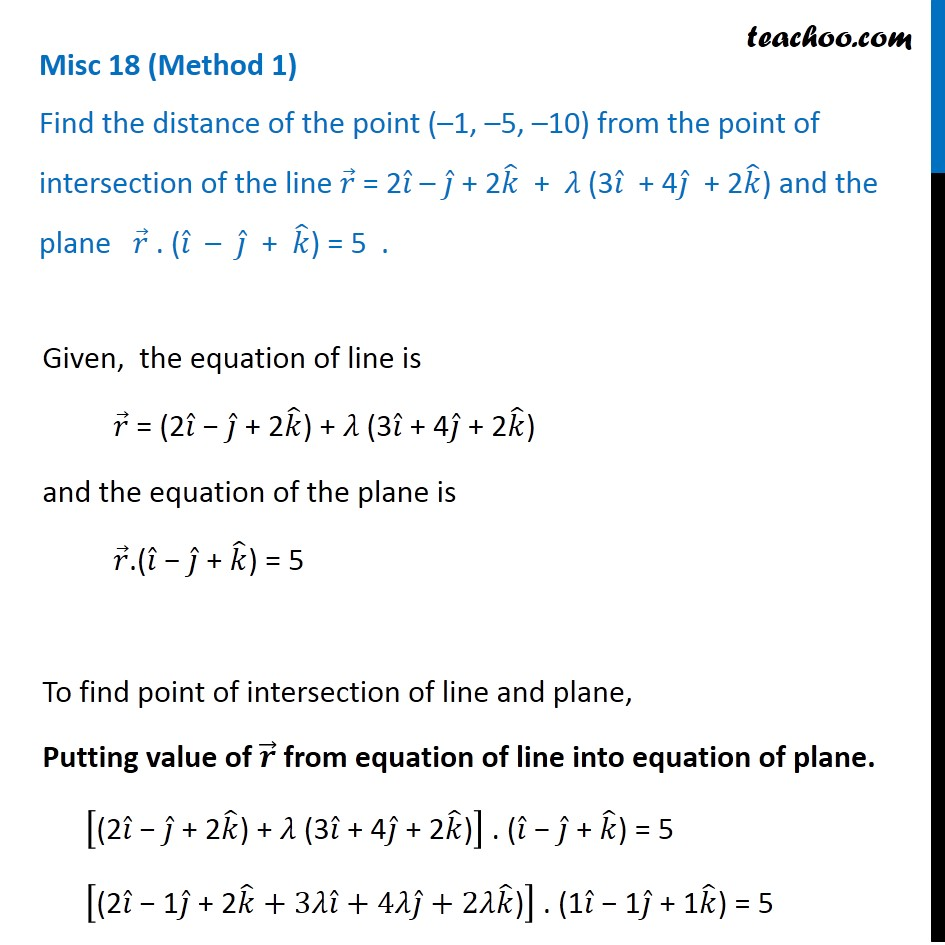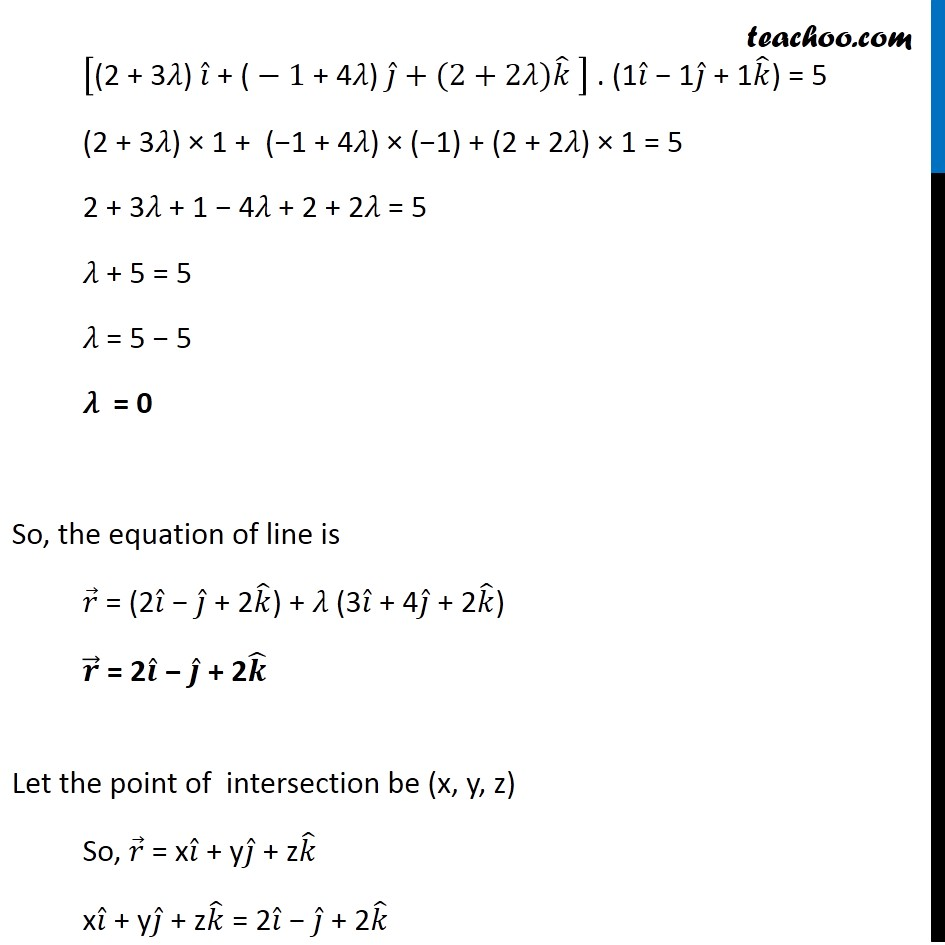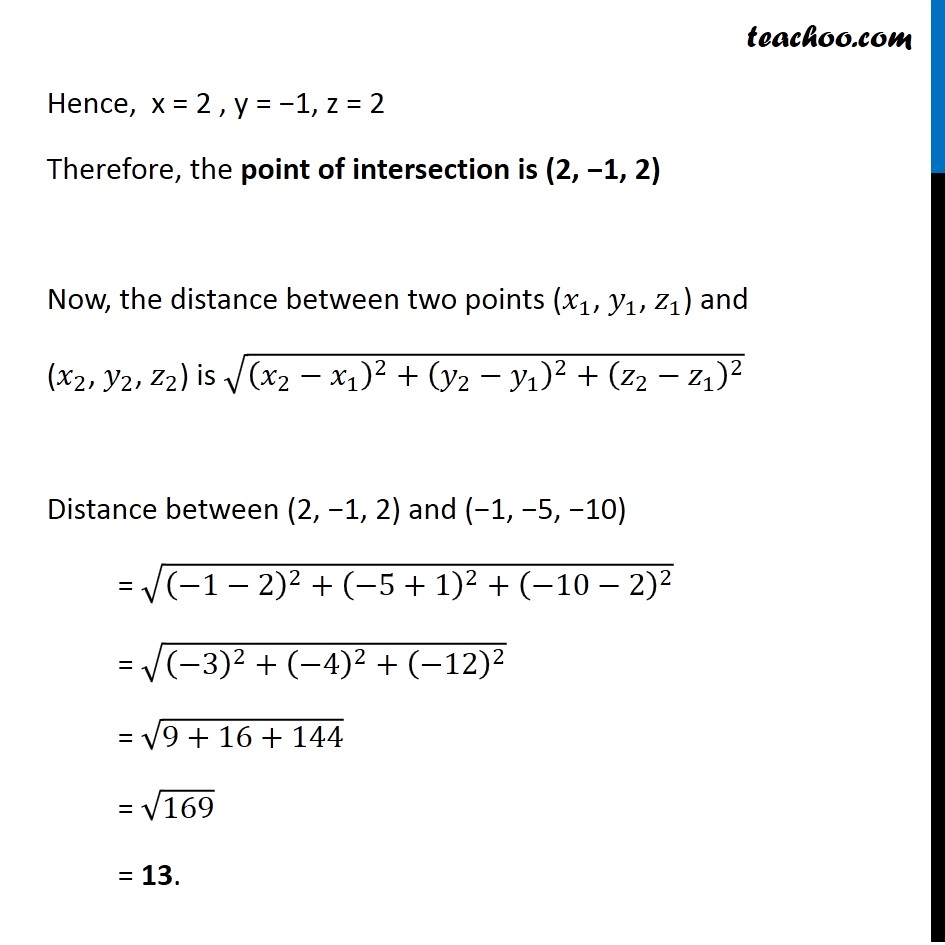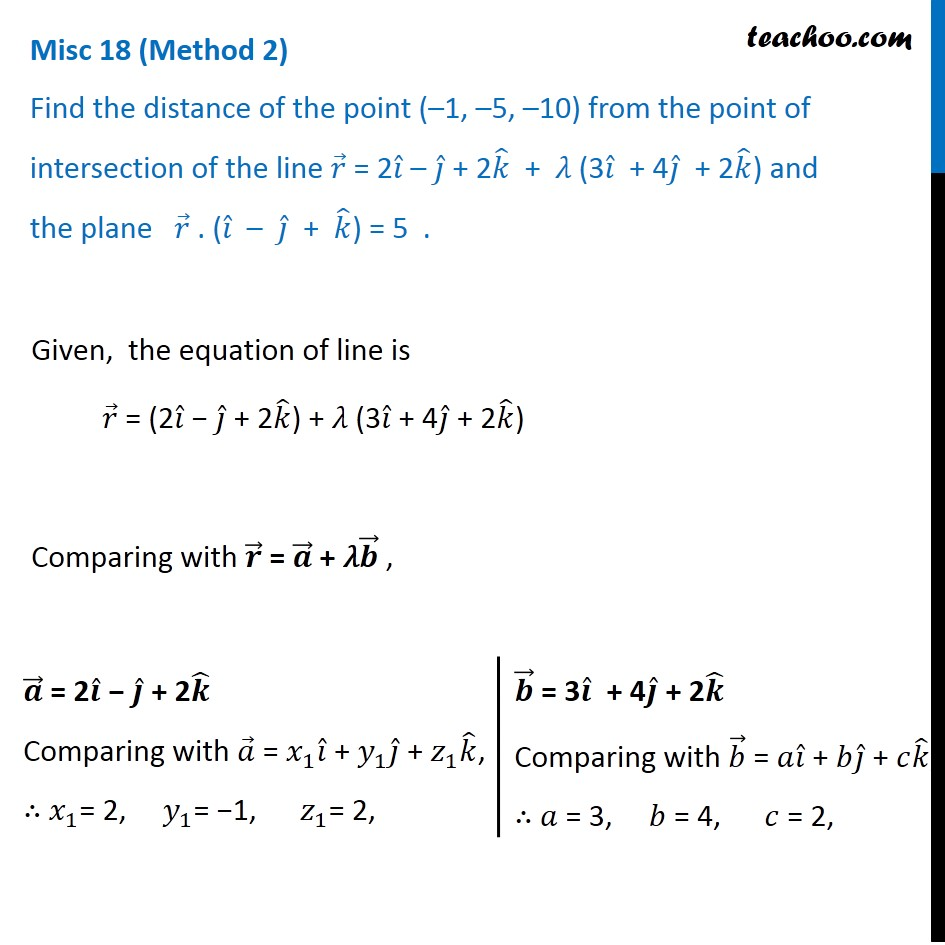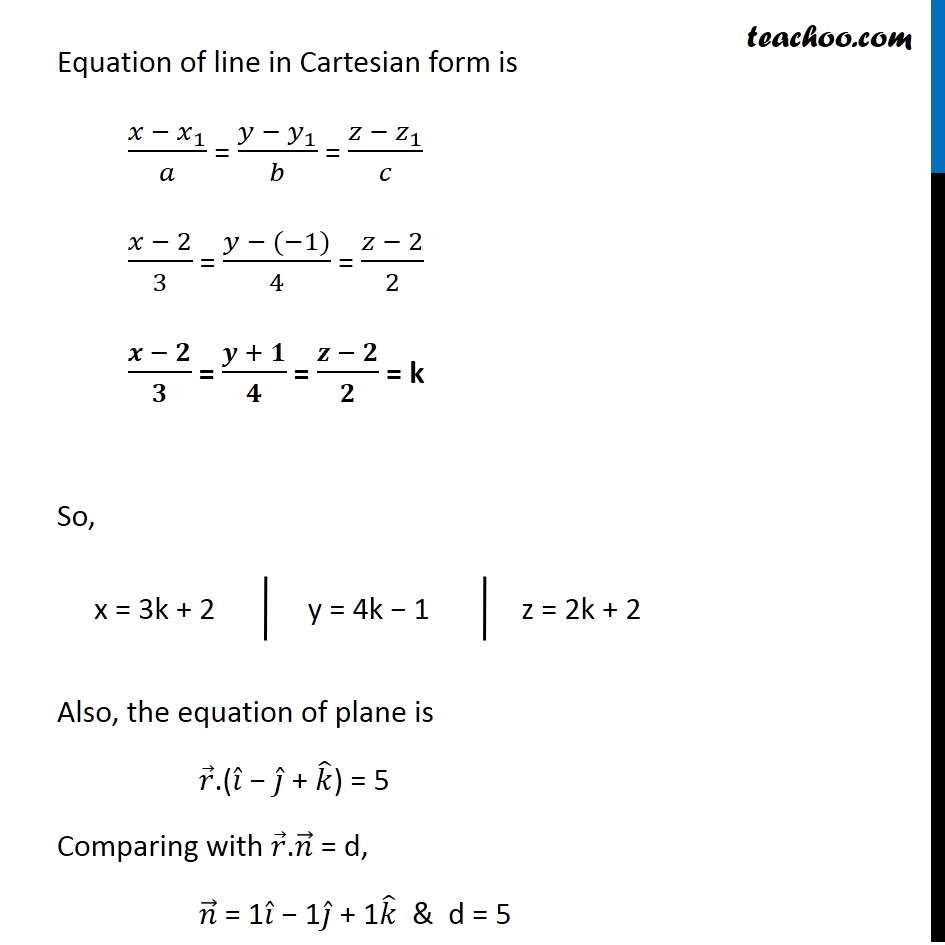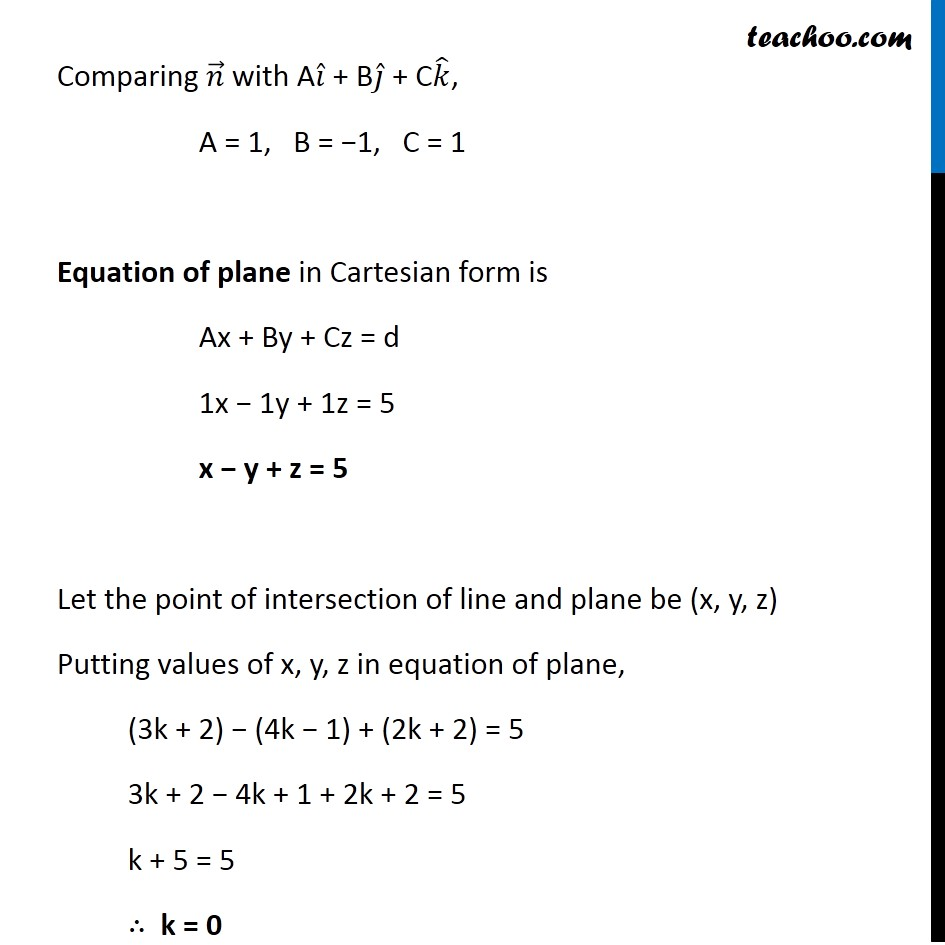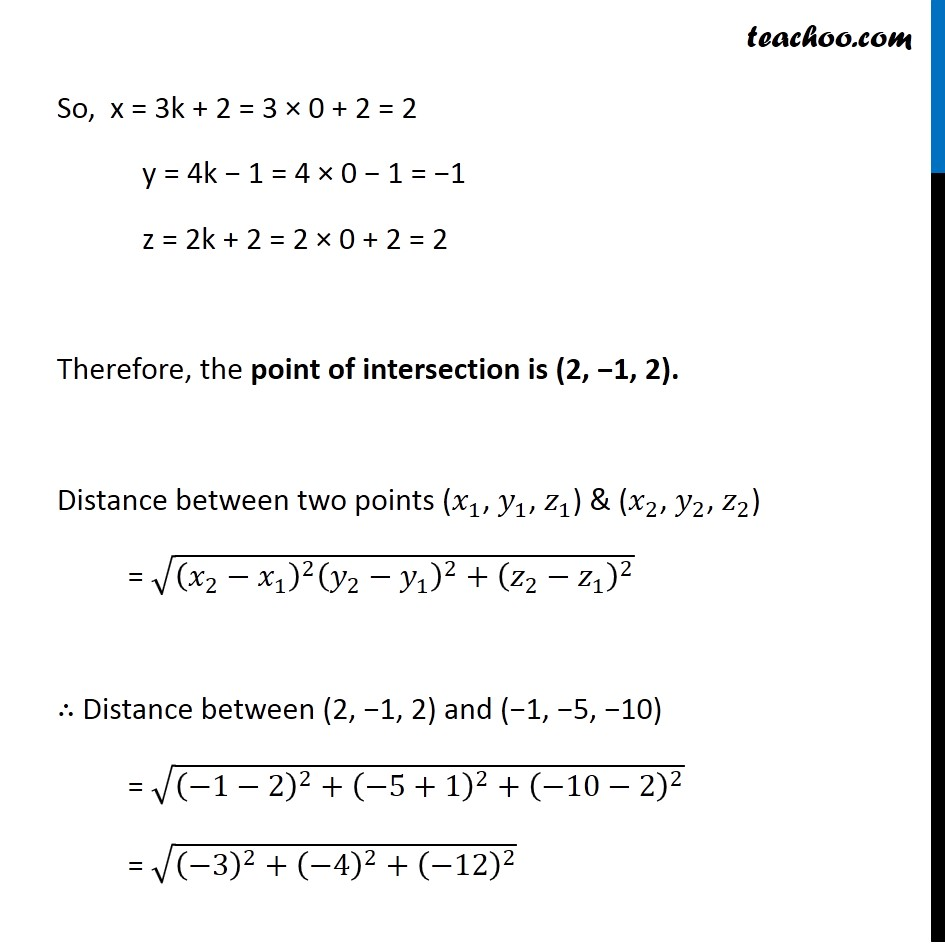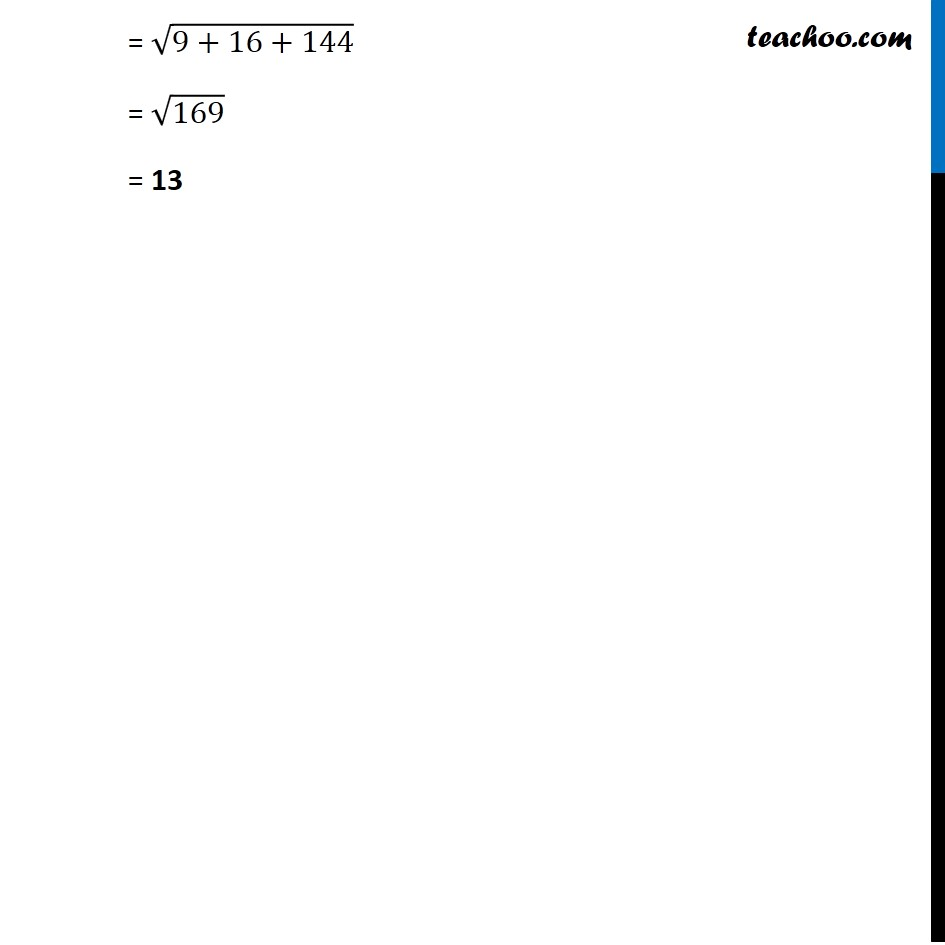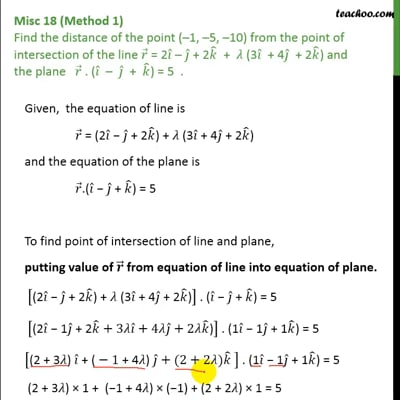This video is only available for Teachoo black users

Introducing your new favourite teacher - Teachoo Black, at only ₹83 per month

### Transcript

Misc 18 (Method 1) Find the distance of the point (–1, –5, –10) from the point of intersection of the line 𝑟 ⃗ = 2𝑖 ̂ – 𝑗 ̂ + 2𝑘 ̂ + 𝜆 (3𝑖 ̂ + 4𝑗 ̂ + 2𝑘 ̂) and the plane 𝑟 ⃗ . (𝑖 ̂ – 𝑗 ̂ + 𝑘 ̂) = 5 .Given, the equation of line is 𝑟 ⃗ = (2𝑖 ̂ − 𝑗 ̂ + 2𝑘 ̂) + 𝜆 (3𝑖 ̂ + 4𝑗 ̂ + 2𝑘 ̂) and the equation of the plane is 𝑟 ⃗.(𝑖 ̂ − 𝑗 ̂ + 𝑘 ̂) = 5 To find point of intersection of line and plane, Putting value of 𝒓 ⃗ from equation of line into equation of plane. ["(2" 𝑖 ̂" − " 𝑗 ̂" + 2" 𝑘 ̂") + 𝜆 (3" 𝑖 ̂" + 4" 𝑗 ̂" + 2" 𝑘 ̂")" ] . (𝑖 ̂ − 𝑗 ̂ + 𝑘 ̂) = 5 ["(2" 𝑖 ̂" − 1" 𝑗 ̂" + 2" 𝑘 ̂+3"𝜆" 𝑖 ̂" + 4𝜆" 𝑗 ̂+2"𝜆" 𝑘 ̂")" ] . (1𝑖 ̂ − 1𝑗 ̂ + 1𝑘 ̂) = 5 ["(2 + 3𝜆) " 𝑖 ̂" + (" −1" + 4𝜆) " 𝑗 ̂+(2+2"𝜆" )𝑘 ̂ ] . (1𝑖 ̂ − 1𝑗 ̂ + 1𝑘 ̂) = 5 (2 + 3𝜆) × 1 + (−1 + 4𝜆) × (−1) + (2 + 2𝜆) × 1 = 5 2 + 3𝜆 + 1 − 4𝜆 + 2 + 2𝜆 = 5 𝜆 + 5 = 5 𝜆 = 5 − 5 𝜆 = 0 So, the equation of line is 𝑟 ⃗ = (2𝑖 ̂ − 𝑗 ̂ + 2𝑘 ̂) + 𝜆 (3𝑖 ̂ + 4𝑗 ̂ + 2𝑘 ̂) 𝒓 ⃗ = 2𝒊 ̂ − 𝒋 ̂ + 2𝒌 ̂ Let the point of intersection be (x, y, z) So, 𝑟 ⃗ = x𝑖 ̂ + y𝑗 ̂ + z𝑘 ̂ x𝑖 ̂ + y𝑗 ̂ + z𝑘 ̂ = 2𝑖 ̂ − 𝑗 ̂ + 2𝑘 ̂ Hence, x = 2 , y = −1, z = 2 Therefore, the point of intersection is (2, −1, 2) Now, the distance between two points (𝑥_1, 𝑦_1, 𝑧_1) and (𝑥_2, 𝑦_2, 𝑧_2) is √((𝑥_2−𝑥_1 )^2 〖+ (𝑦_2−𝑦_1 )〗^2+ (𝑧_2−𝑧_1 )^2 ) Distance between (2, −1, 2) and (−1, −5, −10) = √((−1−2)^2 〖+ (−5+1)〗^2+ (−10−2)^2 ) = √((−3)^2 〖+ (−4)〗^2+ (−12)^2 ) = √(9+16+144) = √169 = 13. Misc 18 (Method 2) Find the distance of the point (–1, –5, –10) from the point of intersection of the line 𝑟 ⃗ = 2𝑖 ̂ – 𝑗 ̂ + 2𝑘 ̂ + 𝜆 (3𝑖 ̂ + 4𝑗 ̂ + 2𝑘 ̂) and the plane 𝑟 ⃗ . (𝑖 ̂ – 𝑗 ̂ + 𝑘 ̂) = 5 .Given, the equation of line is 𝑟 ⃗ = (2𝑖 ̂ − 𝑗 ̂ + 2𝑘 ̂) + 𝜆 (3𝑖 ̂ + 4𝑗 ̂ + 2𝑘 ̂) Comparing with 𝒓 ⃗ = 𝒂 ⃗ + 𝜆𝒃 ⃗ , 𝒂 ⃗ = 2𝒊 ̂ − 𝒋 ̂ + 2𝒌 ̂ Comparing with 𝑎 ⃗ = 𝑥_1 𝑖 ̂ + 𝑦_1 𝑗 ̂ + 𝑧_1 𝑘 ̂, ∴ 𝑥_1= 2, 𝑦_1= −1, 𝑧_1= 2, 𝒃 ⃗ = 3𝒊 ̂ + 4𝒋 ̂ + 2𝒌 ̂ Comparing with 𝑏 ⃗ = 𝑎𝑖 ̂ + 𝑏𝑗 ̂ + 𝑐𝑘 ̂, ∴ 𝑎 = 3, 𝑏 = 4, 𝑐 = 2, Equation of line in Cartesian form is (𝑥 − 𝑥_1)/𝑎 = (𝑦 − 𝑦_1)/𝑏 = (𝑧 − 𝑧_1)/𝑐 (𝑥 − 2)/3 = (𝑦 − (−1))/4 = (𝑧 − 2)/2 (𝒙 − 𝟐)/𝟑 = (𝒚 + 𝟏)/𝟒 = (𝒛 − 𝟐)/𝟐 = k So, Also, the equation of plane is 𝑟 ⃗.(𝑖 ̂ − 𝑗 ̂ + 𝑘 ̂) = 5 Comparing with 𝑟 ⃗.𝑛 ⃗ = d, 𝑛 ⃗ = 1𝑖 ̂ − 1𝑗 ̂ + 1𝑘 ̂ & d = 5 Comparing 𝑛 ⃗ with A𝑖 ̂ + B𝑗 ̂ + C𝑘 ̂, A = 1, B = −1, C = 1 Equation of plane in Cartesian form is Ax + By + Cz = d 1x − 1y + 1z = 5 x − y + z = 5 Let the point of intersection of line and plane be (x, y, z) Putting values of x, y, z in equation of plane, (3k + 2) − (4k − 1) + (2k + 2) = 5 3k + 2 − 4k + 1 + 2k + 2 = 5 k + 5 = 5 ∴ k = 0 So, x = 3k + 2 = 3 × 0 + 2 = 2 y = 4k − 1 = 4 × 0 − 1 = −1 z = 2k + 2 = 2 × 0 + 2 = 2 Therefore, the point of intersection is (2, −1, 2). Distance between two points (𝑥_1, 𝑦_1, 𝑧_1) & (𝑥_2, 𝑦_2, 𝑧_2) = √((𝑥_2−𝑥_1 )^2 (𝑦_2−𝑦_1 )^2+(𝑧_2−𝑧_1 )^2 ) ∴ Distance between (2, −1, 2) and (−1, −5, −10) = √((−1−2)^2+(−5+1)^2+(−10−2)^2 ) = √((−3)^2+(−4)^2+(−12)^2 ) = √(9+16+144) = √169 = 13